# NCERT Solutions for Class 9 Maths Chapter 9 Exercise 9.2

Download NCERT Solutions for Class 9 Maths Chapter 9 Exercise 9.2 – Areas of Parallelograms And Triangles. This Exercise contains 6 questions, for which detailed answers have been provided in this note. In case you are looking at studying the remaining Exercise for Class 9 for Maths NCERT solutions for Chapter 9 or other Chapters, you can click the link at the end of this Note.

### NCERT Solutions for Class 9 Maths Chapter 9 Exercise 9.2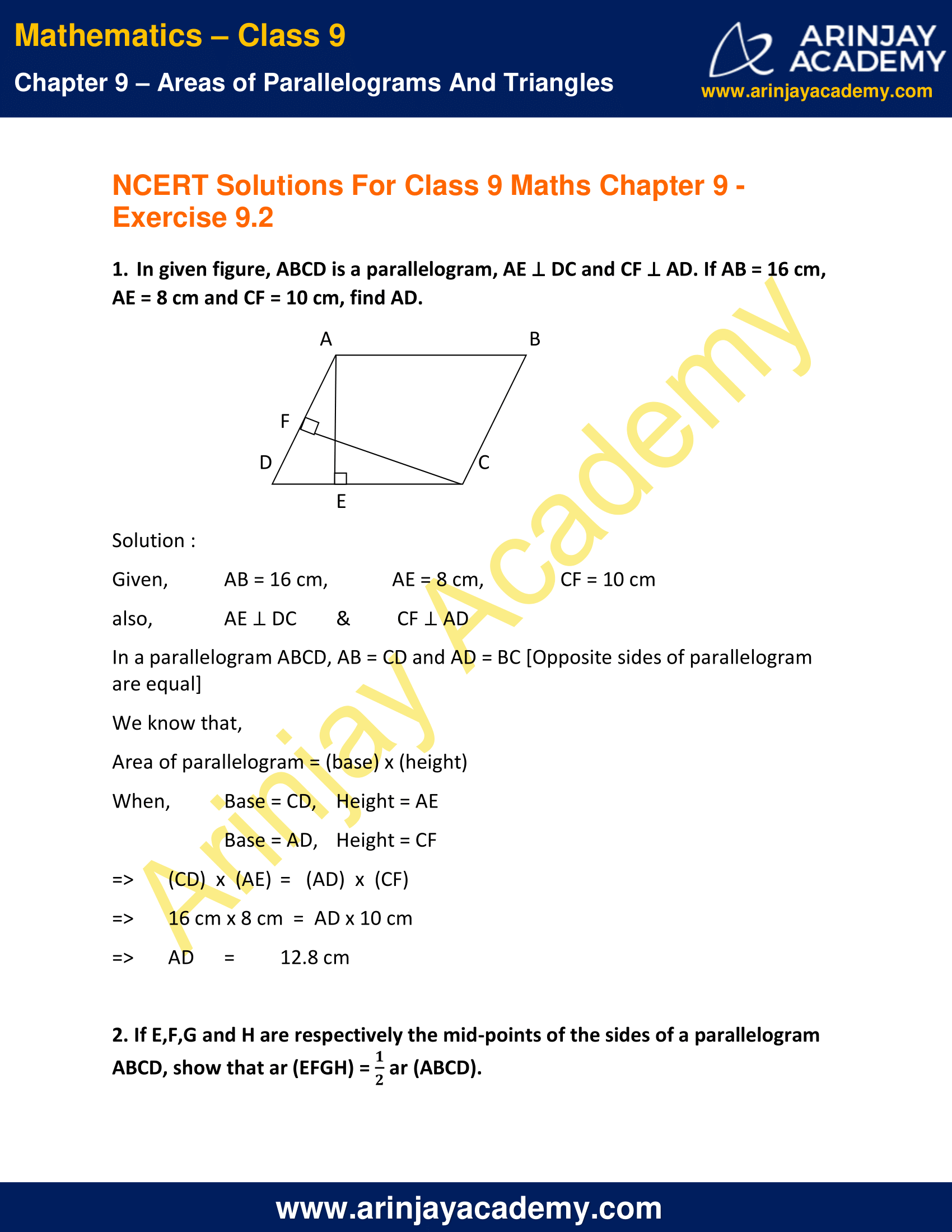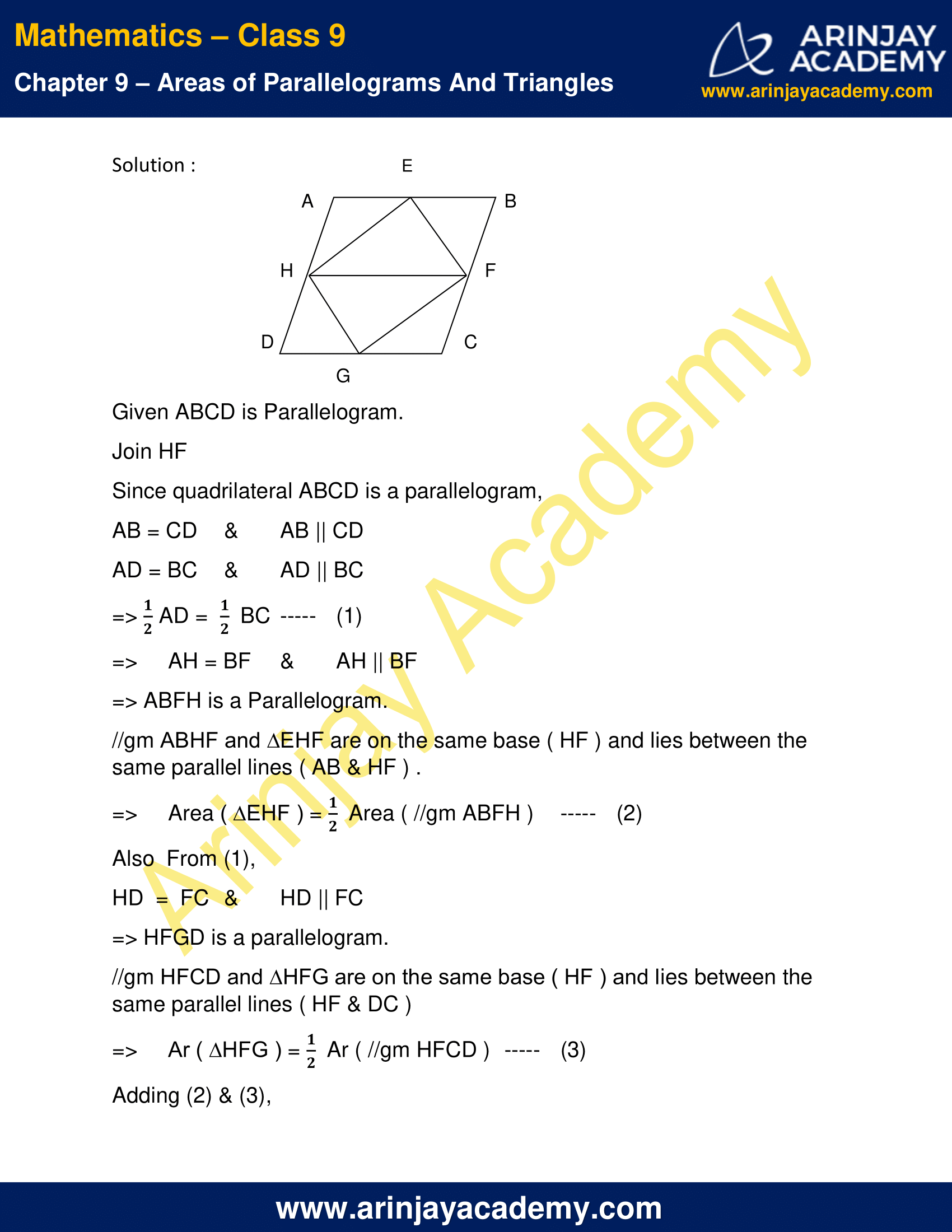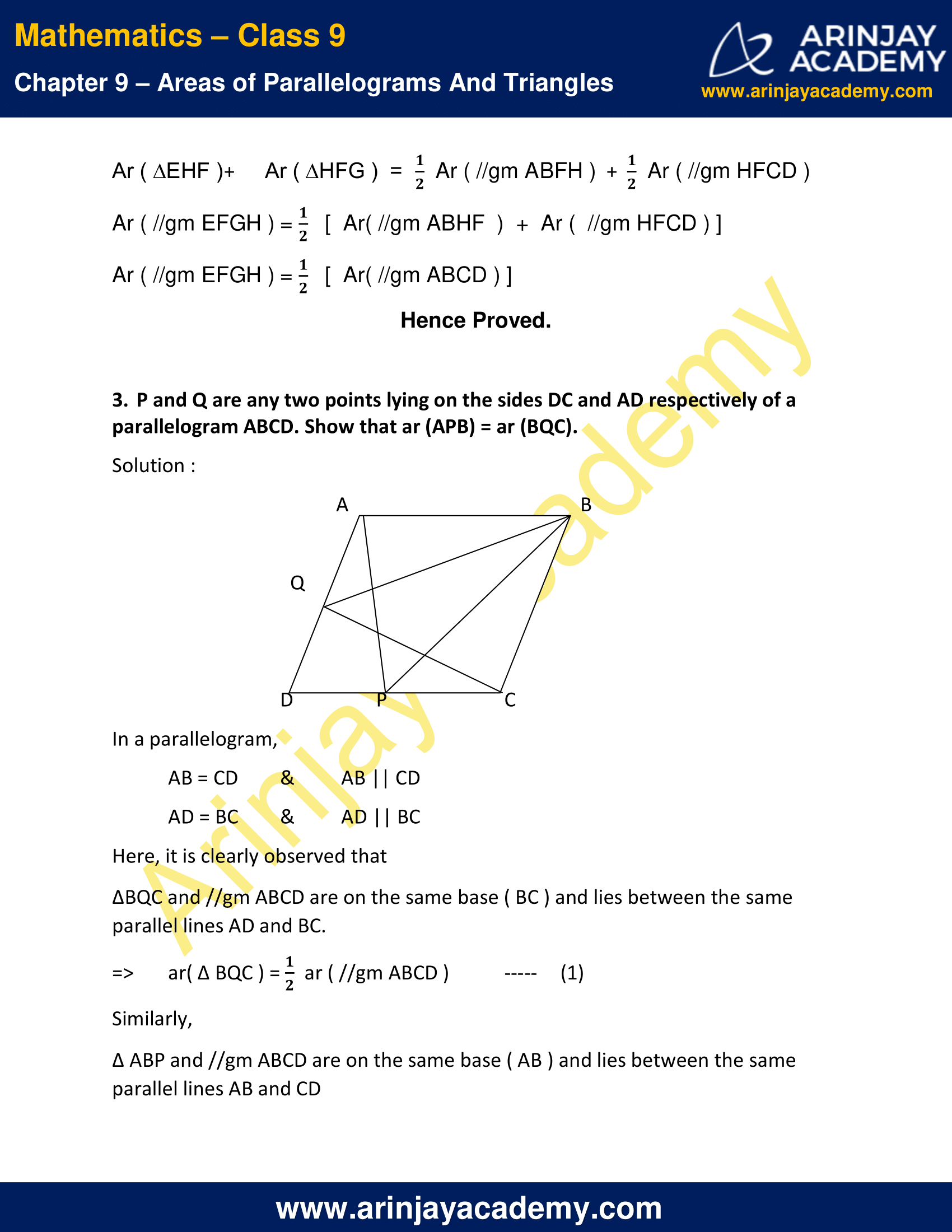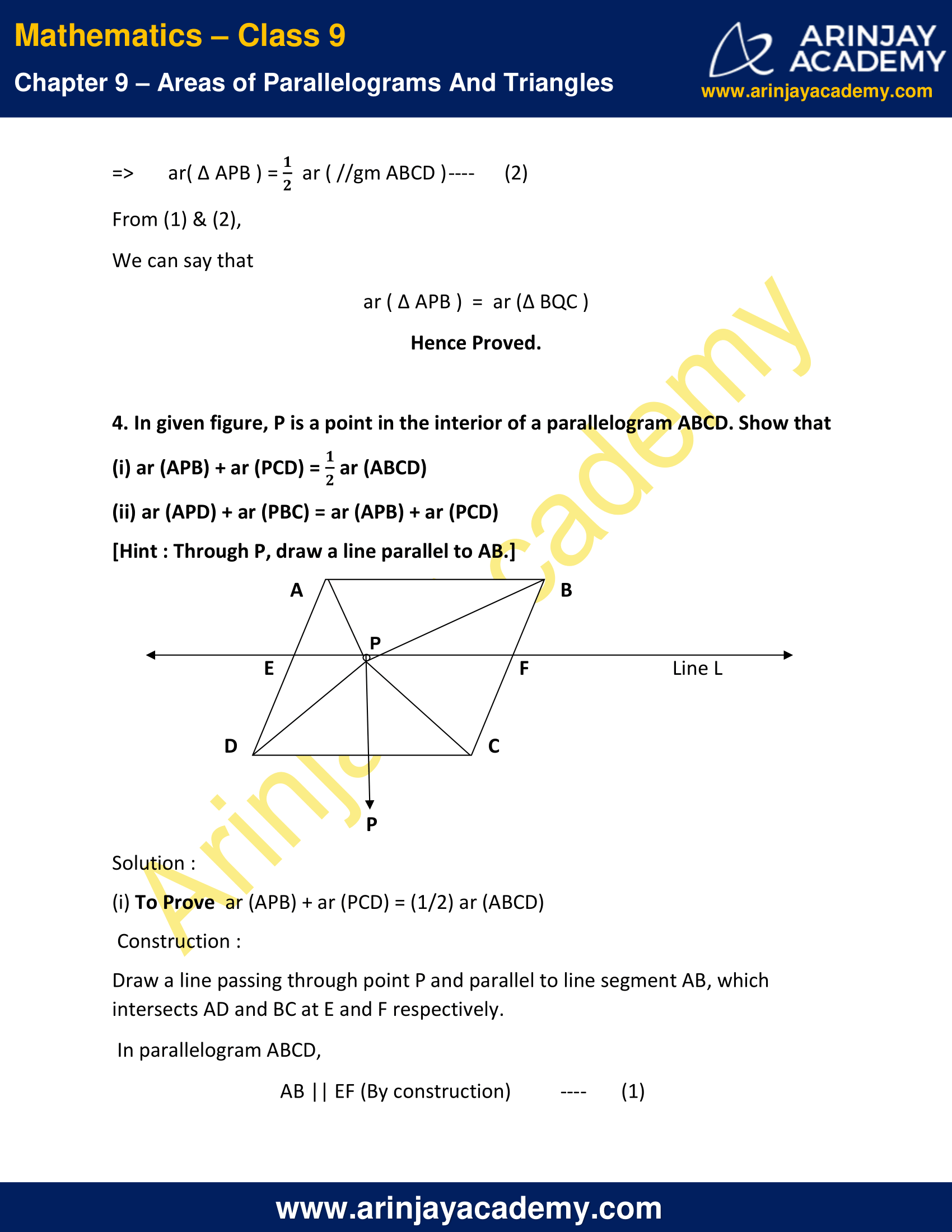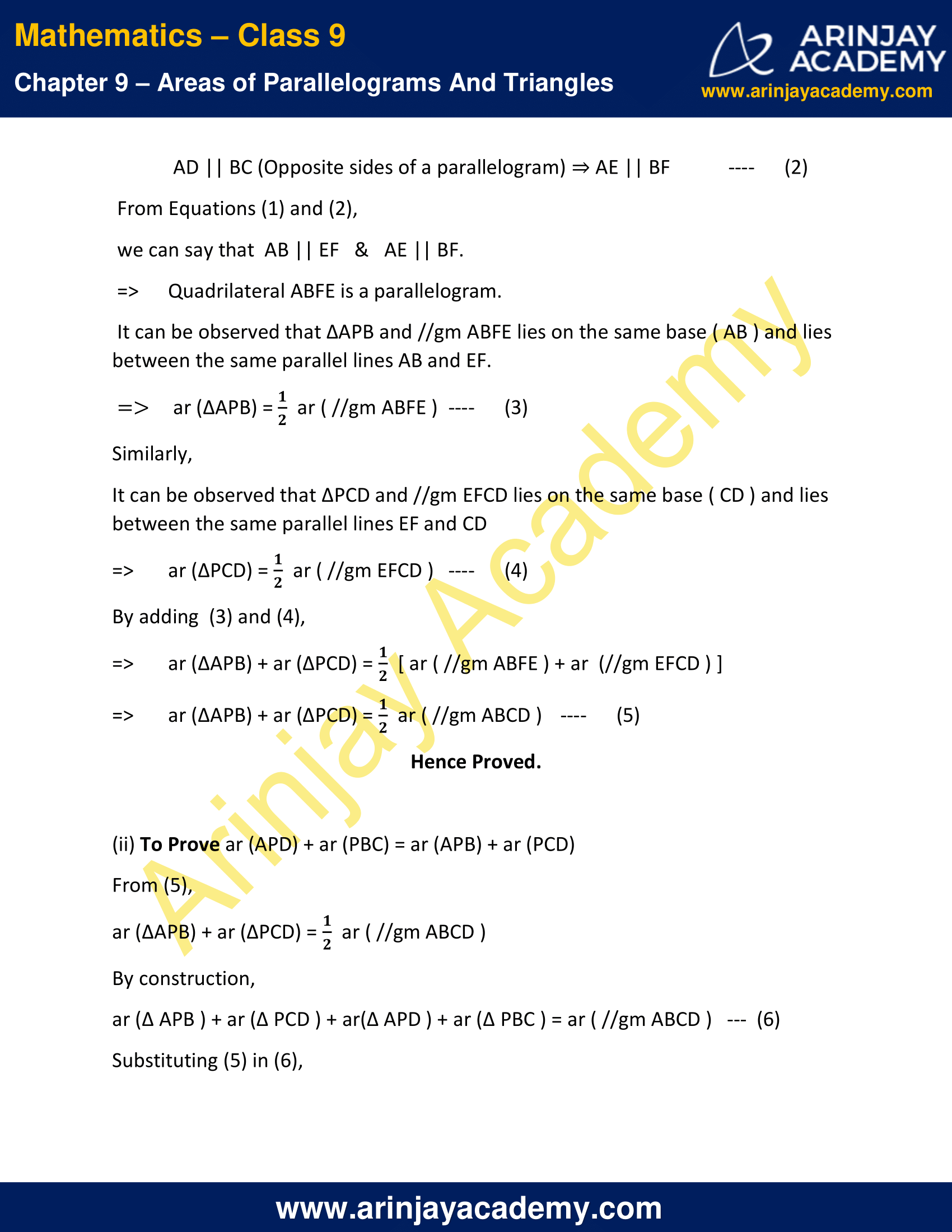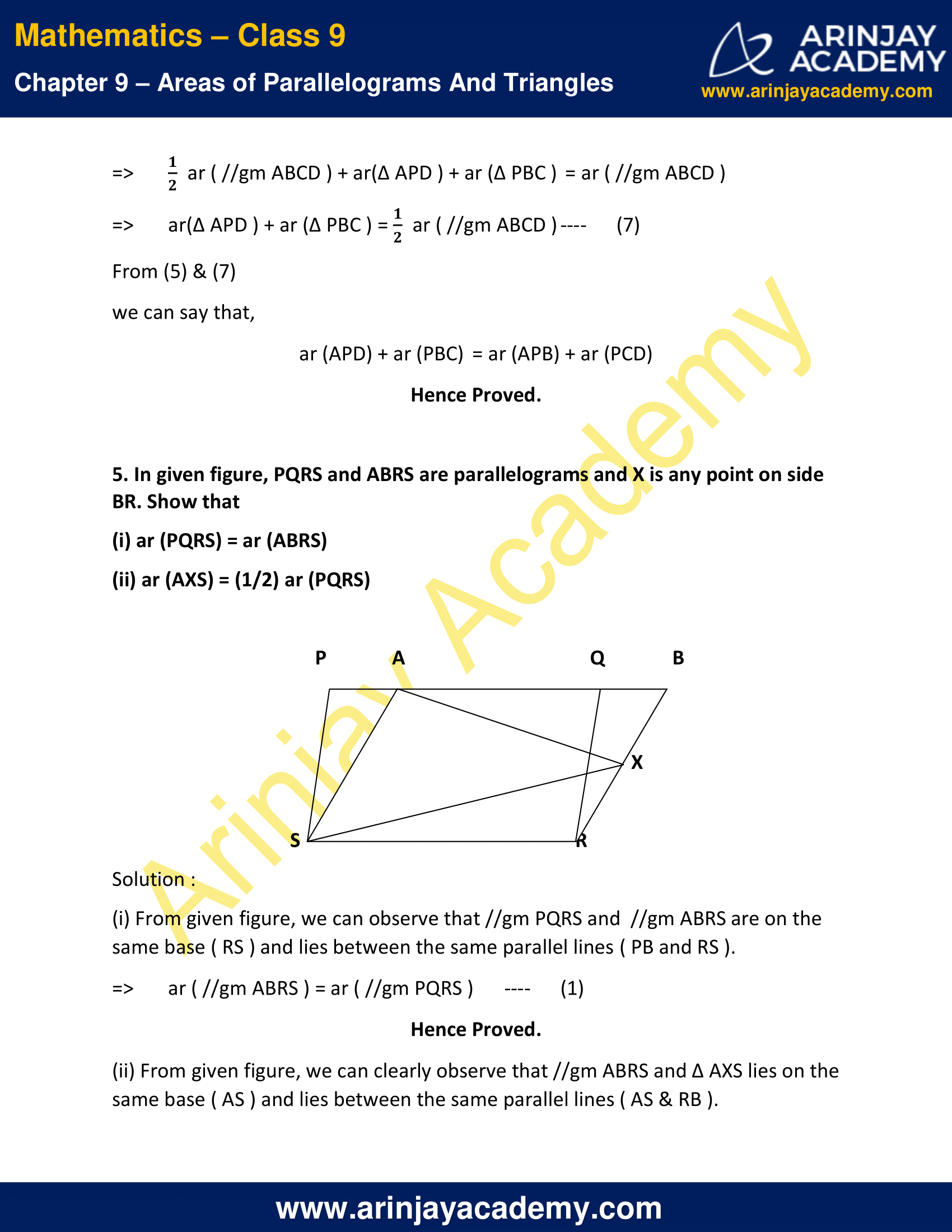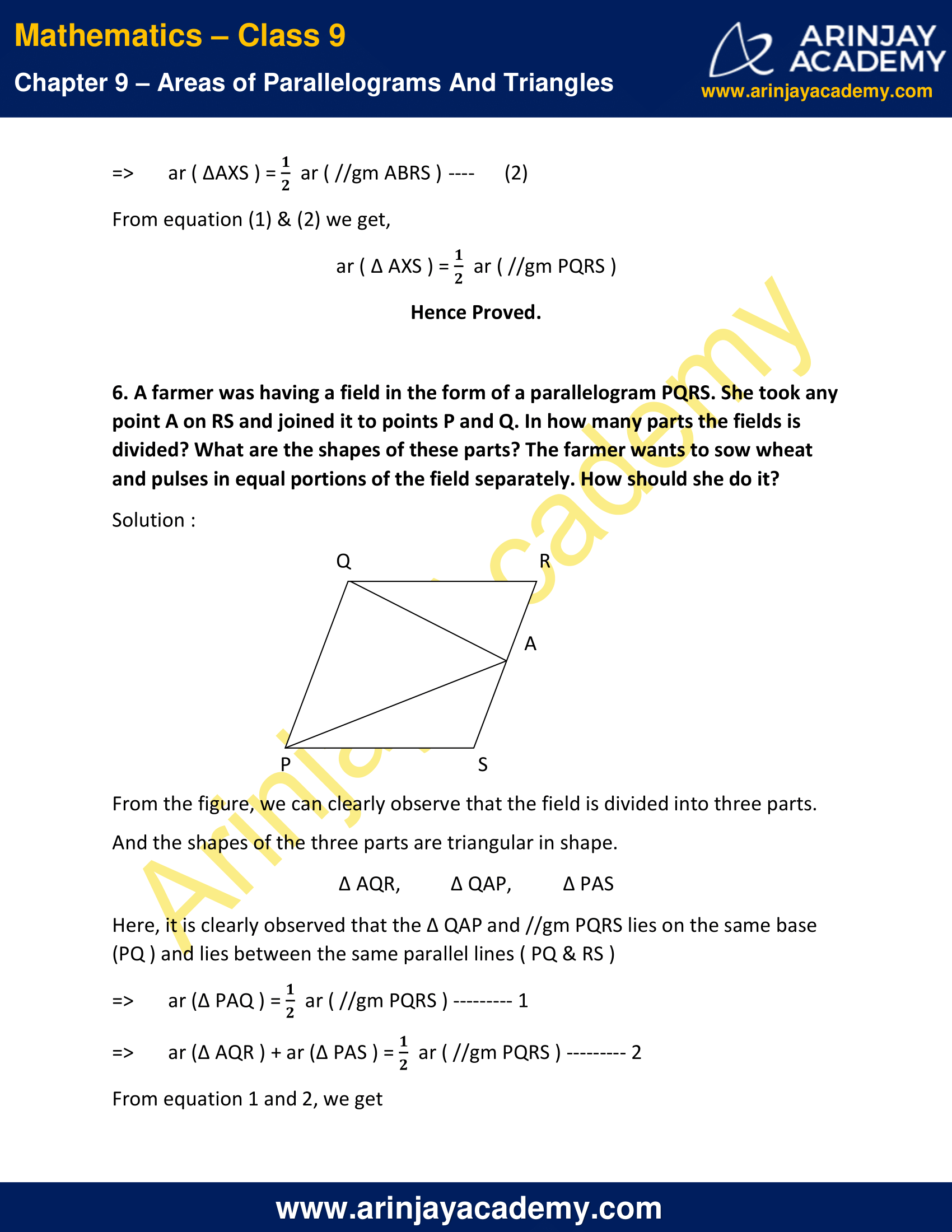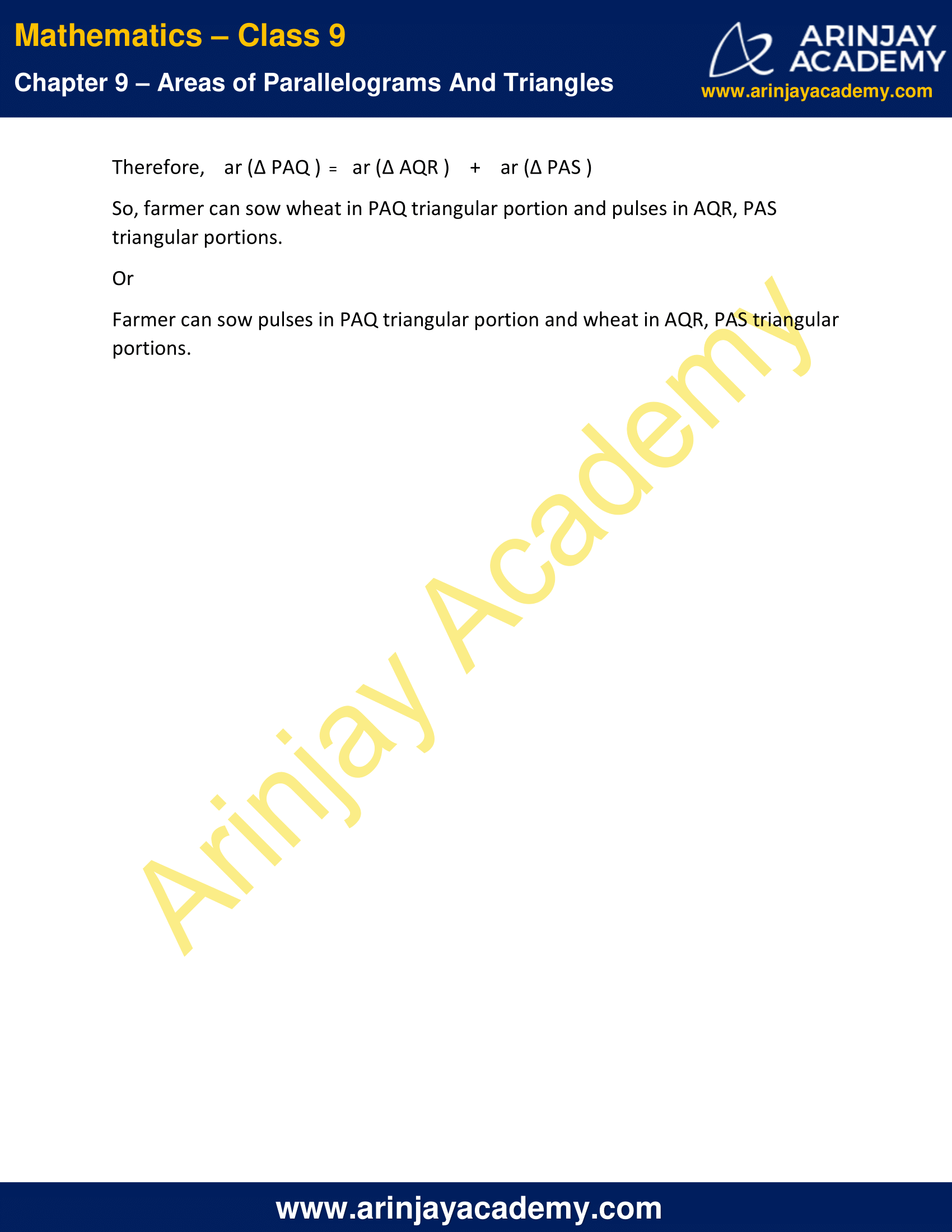NCERT Solutions for Class 9 Maths Chapter 9 Exercise 9.2

1. In given figure, ABCD is a parallelogram, AE DC and CF AD. If AB = 16 cm, AE = 8 cm and CF = 10 cm, find AD.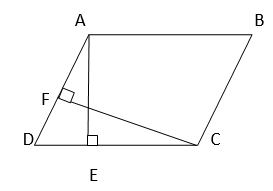Solution :

Given:
AB = 16 cm
AE = 8 cm
CF = 10 cm
AE ⊥ DC
CF ⊥ AD

In a parallelogram ABCD, AB = CD and AD = BC [Opposite sides of parallelogram are equal]
We know that,
Area of parallelogram = (base) x (height)
When, Base = CD,    Height = AE
Base = AD,    Height = CF
=> (CD)  x  (AE)  =   (AD)  x  (CF)
=> 16 cm x 8 cm  =  AD x 10 cm
=> AD = 12.8 cm
AD = 12.8 cm

2. If E,F,G and H are respectively the mid-points of the sides of a parallelogram ABCD, show that ar (EFGH) = 1/2 ar (ABCD).

Solution :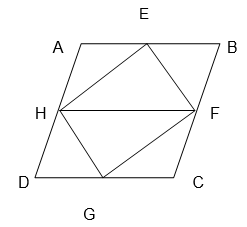Given ABCD is Parallelogram.
Join HF
Since quadrilateral ABCD is a parallelogram,
AB = CD & AB || CD
AD = BC & AD || BC
=> 1/2 AD = 1/2 BC —–    (1)
=>     AH = BF & AH || BF
=> ABFH is a Parallelogram.
//gm ABHF and ∆EHF are on the same base ( HF ) and lies between the same parallel lines ( AB & HF ) .
=>     Area ( ∆EHF ) = 1/2 Area ( //gm ABFH )    —–    (2)
Also  From (1),
HD  =  FC & HD || FC
=> HFGD is a parallelogram.
//gm HFCD and ∆HFG are on the same base ( HF ) and lies between the same parallel lines ( HF & DC )
=>     Ar ( ∆HFG ) = 1/2 Ar ( //gm HFCD )  —–    (3)
Adding (2) & (3),
Ar ( ∆EHF ) +  Ar ( ∆HFG )  =  1/2 Ar ( //gm ABFH ) + 1/2 Ar ( //gm HFCD )
Ar ( //gm EFGH ) = 1/2 [  Ar( //gm ABHF  )  +  Ar (  //gm HFCD ) ]
Ar ( //gm EFGH ) = 1/2 [  Ar( //gm ABCD ) ]
Hence Proved.

3. P and Q are any two points lying on the sides DC and AD respectively of a parallelogram ABCD. Show that ar (APB) = ar (BQC).

Solution :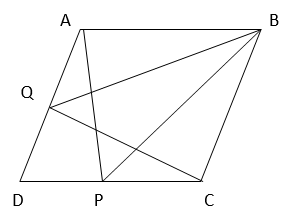In a parallelogram,
AB = CD & AB || CD
AD = BC & AD || BC
Here, it is clearly observed that
∆BQC and //gm ABCD are on the same base ( BC ) and lies between the same parallel lines AD and BC.
=> ar( ∆ BQC ) = 1/2 ar ( //gm ABCD )   ….(1)
Similarly,
∆ ABP and //gm ABCD are on the same base ( AB ) and lies between the same parallel lines AB and CD
=> ar( ∆ APB ) = 1/2 ar ( //gm ABCD )   ….(2)
From (1) & (2),
We can say that
ar ( ∆ APB )  =  ar (∆ BQC )
Hence Proved.

4. In given figure, P is a point in the interior of a parallelogram ABCD. Show that

(i) ar (APB) + ar (PCD) = 1/2 ar (ABCD)

(ii) ar (APD) + ar (PBC) = ar (APB) + ar (PCD)

[Hint : Through P, draw a line parallel to AB.]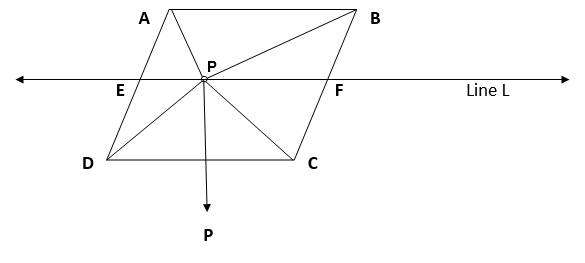Solution :

(i) To Prove  ar (APB) + ar (PCD) = (1/2) ar (ABCD)
Construction :
Draw a line passing through point P and parallel to line segment AB, which intersects AD and BC at E and F respectively.
In parallelogram ABCD,
AB || EF (By construction) —- (1)
AD || BC (Opposite sides of a parallelogram) ⇒ AE || BF —- (2)
From Equations (1) and (2), we can say that  AB || EF   &   AE || BF.
=> Quadrilateral ABFE is a parallelogram.
It can be observed that ∆APB and //gm ABFE lies on the same base ( AB ) and lies between the same parallel lines AB and EF.
=>    ar (∆APB) = 1/2 ar ( //gm ABFE ) — (3)
Similarly,
It can be observed that ∆PCD and //gm EFCD lies on the same base ( CD ) and lies between the same parallel lines EF and CD
=> ar (∆PCD) = 1/2 ar ( //gm EFCD )   —-      (4)
By adding  (3) and (4),
=> ar (∆APB) + ar (∆PCD) = 1/2 [ ar ( //gm ABFE ) + ar  (//gm EFCD ) ]
=> ar (∆APB) + ar (∆PCD) = 1/2 ar ( //gm ABCD )    —-      (5)

Hence Proved.

(ii) To Prove ar (APD) + ar (PBC) = ar (APB) + ar (PCD)
From (5),
ar (∆APB) + ar (∆PCD) = 1/2 ar ( //gm ABCD )
By construction,
ar (∆ APB ) + ar (∆ PCD ) + ar(∆ APD ) + ar (∆ PBC ) = ar ( //gm ABCD )   —  (6)
Substituting (5) in (6),
=> 1/2 ar ( //gm ABCD ) + ar(∆ APD ) + ar (∆ PBC ) = ar ( //gm ABCD )
=> ar(∆ APD ) + ar (∆ PBC ) = 1/2 ar ( //gm ABCD ) —-      (7)
From (5) & (7)
we can say that,
ar (APD) + ar (PBC) = ar (APB) + ar (PCD)
Hence Proved.

5. In given figure, PQRS and ABRS are parallelograms and X is any point on side BR. Show that
(i) ar (PQRS) = ar (ABRS)
(ii) ar (AXS) = (1/2) ar (PQRS)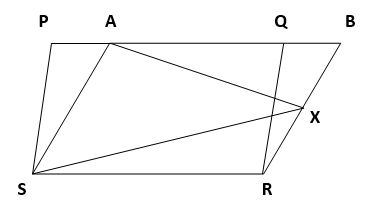Solution :

(i) From given figure, we can observe that //gm PQRS and  //gm ABRS are on the same base ( RS ) and lies between the same parallel lines ( PB and RS ).
=> ar ( //gm ABRS ) = ar ( //gm PQRS ) —- (1)
Hence Proved.

(ii) From given figure, we can clearly observe that //gm ABRS and ∆ AXS lies on the same base ( AS ) and lies between the same parallel lines ( AS & RB ).
=> ar ( ∆AXS ) = 1/2 ar ( //gm ABRS ) —-      (2)
From equation (1) & (2) we get,
ar ( ∆ AXS ) = 1/2 ar ( //gm PQRS )
Hence Proved.

6. A farmer was having a field in the form of a parallelogram PQRS. She took any point A on RS and joined it to points P and Q. In how many parts the fields is divided? What are the shapes of these parts? The farmer wants to sow wheat and pulses in equal portions of the field separately. How should she do it?

Solution :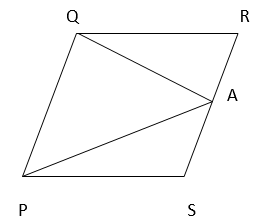From the figure, we can clearly observe that the field is divided into three parts.
And the shapes of the three parts are triangular in shape.
∆ AQR, ∆ QAP, ∆ PAS
Here, it is clearly observed that the ∆ QAP and //gm PQRS lies on the same base (PQ ) and lies between the same parallel lines ( PQ & RS )
=> ar (∆ PAQ ) =  1/2 ar ( //gm PQRS ) ——— 1
=> ar (∆ AQR ) + ar (∆ PAS ) = 1/2 ar ( //gm PQRS ) ——— 2
From equation 1 and 2, we get
Therefore,    ar (∆ PAQ )  =    ar (∆ AQR )    +    ar (∆ PAS )
So, farmer can sow wheat in PAQ triangular portion and pulses in AQR, PAS triangular portions.
Or
Farmer can sow pulses in PAQ triangular portion and wheat in AQR, PAS triangular portions.

NCERT Solutions for Class 9 Maths Chapter 9 Exercise 9.2 – Areas of Parallelograms And Triangles, has been designed by the NCERT to test the knowledge of the student on the topic – Parallelograms on the same Base and Between the same Parallels

The next Exercise for NCERT Solutions for Class 9 Maths Chapter 9 Exercise 9.3 – Areas of Parallelograms And Triangles can be accessed by clicking here.

Download NCERT Solutions for Class 9 Maths Chapter 9 Exercise 9.2 – Areas of Parallelograms And Triangles